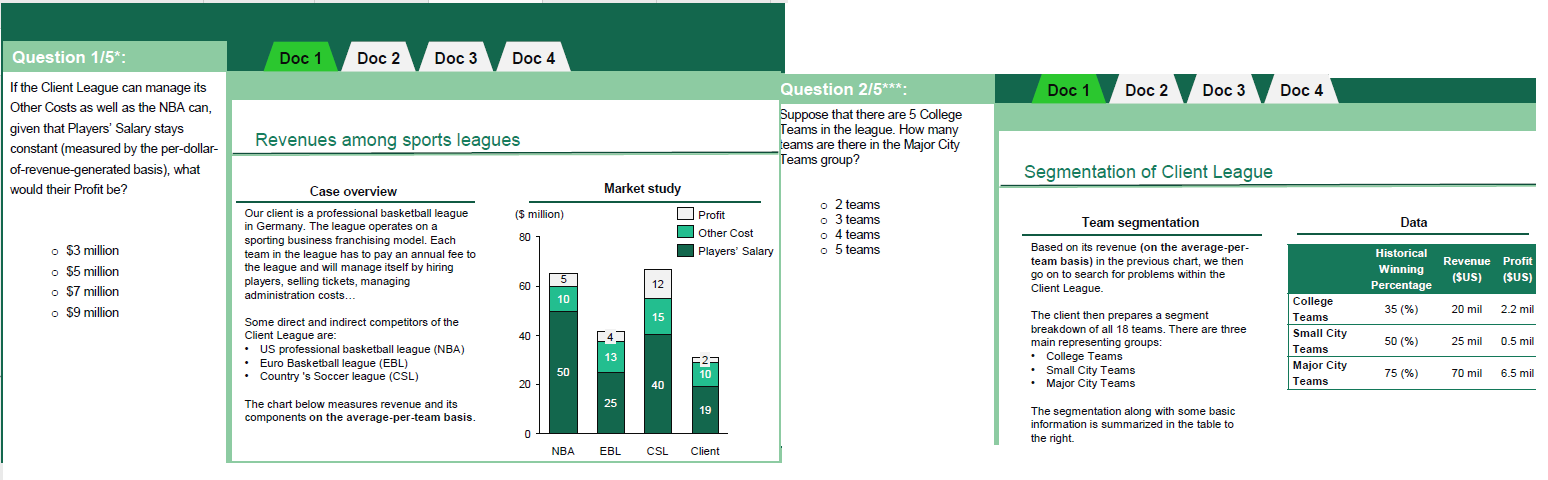# BCG Potential Test Sample Question

BCG BCG Potential Test Potential Test
New answer on Jul 02, 2021
1.3 k Views

Hello everyone!

Today I have been doing some practices regarding the BCG Potential Test, however I found the following questions which I don't know how to solve it.Thank you very much.

(edited)

• Date ascending
• Date descending

Hi Floyd,

In regards to #1 you have to figure out what % of total revenues the "Other Costs" equals. The NBA's other costs are \$10M and they have total revenues of \$65M (5+10+50). As such, their other costs are 15.38%.

If our client could have the same cost structure, their "Other Costs" would be \$4.77 (\$31M times 15.38%). As such, their additional profit would be 5.33 (Current Other Costs of \$10M minus the new costs of \$4.77 = added pure profit).

Adding the additional profit to their existing profit gets us \$7.33M, so the answer is C - \$7M